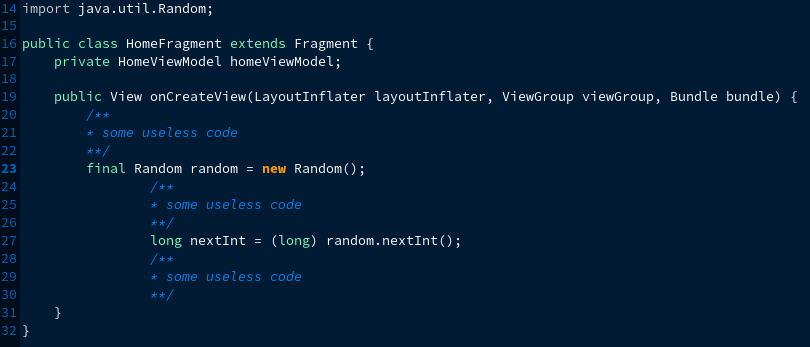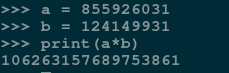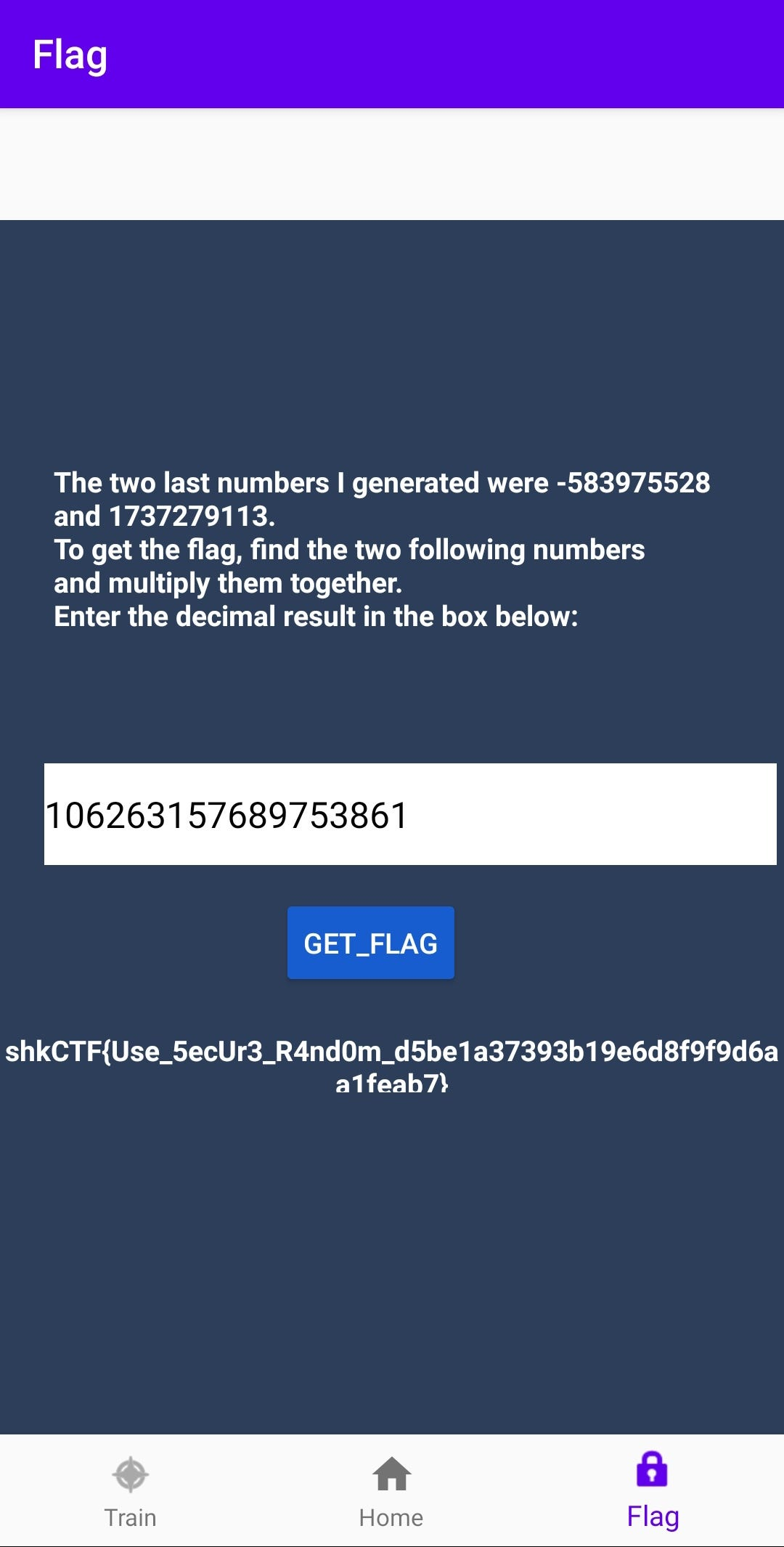# Sharky CTF | Casino

The challenge description goes like this:

``````Get rich.

Creator : \$in
``````

casino_apk

What we have here is a crypto challenge in which we have to:

1. Reverse Engineer the APK using the tool of your choice, I used jadx.

2. Install the APK on our android device or an emulator.

Now using the application I found that it’s asking for the next two numbers when it has given us the first two randomly generated numbers and the application uses Java’s RNG as we can see here in the source code;Now what we need to do is find the seed for the RNG to spit out next Integers for us and as we have the first two integers the becomes even easier to do.

Let’s take out our friend Google or DuckDuckGo [Don’t fight me] ://How quirky, we have exactly what we need in the first link. Someone God-like has posted the whole code that we need to use in the answer.

So now all we need to do is edit the first two numbers provided in this code with the two we have from the source code, -583975528 and 1737279113.

``````import java.util.Random;

public class lol {
// implemented after https://docs.oracle.com/javase/7/docs/api/java/util/Random.html
public static int next(long seed) {
int bits=32;
long seed2 = (seed * 0x5DEECE66DL + 0xBL) & ((1L << 48) - 1);
return (int)(seed2 >>> (48 - bits));
}

public static void main(String[] args) {
System.out.println("Starting");
long i1 = -1952542633L; //change this to -583975528
long i2 = -284611532L; //change this to 1737279113
long seed =0;
for (int i = 0; i < 65536; i++) {
seed = i1 *65536 + i;
if (next(seed) == i2) {
System.out.println("Seed found: " + seed);
break;
}
}
Random random = new Random((seed ^ 0x5DEECE66DL) & ((1L << 48) - 1));
int oUseless = random.nextInt(); //this will spit 1737279113
int o1 = random.nextInt();
int o2 = random.nextInt();

System.out.println("So we have that first next number is: "+o1+" and the second one is: "+o2+" with seed: "+seed);

}
}
``````

That is it, we have both of the numbers now we just have to multiply them and put the result in the casino app.Always use python as a calculator.

Boom! we have a flag.